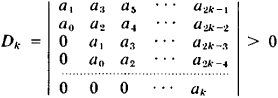# Hurwitz Criterion

The following article is from The Great Soviet Encyclopedia (1979). It might be outdated or ideologically biased.

## Hurwitz Criterion

a criterion that makes it possible to determine when all the roots of the polynomial

p(z) ‗ aozn + a1zn-1 + . . . + an-1z + an

have negative real parts. For example, for the polynomials with real coefficients a0 > 0, a1, . . . ,an, the Hurwitz criterion states the following: in order that all the roots of the polynomial p(z) have negative real parts, it is necessary and sufficient that for all k = 1,2, ... , n the following inequality is valid:This criterion was discovered by the German mathematician A. Hurwitz in 1895. It is used primarily to determine the stability of the solutions of a system of differential equations with constant coefficients.

### REFERENCES

Kurosh, A. G. Kurs vysshei algebry, 9th ed. Moscow-Leningrad. 1968.
Chetaev. N. G. Ustoichivost’ dvizheniia. Moscow-Leningrad, 1946.
References in periodicals archive ?
In order to limit the evaluation value of each individual of the population within a reasonable range, the Routh- Hurwitz criterion must be employed to test the closed-loop system stability before evaluating the evaluation value of an individual.
In the literature, papers that employ adopted Hurwitz criterion for ranking issues can be found [26-28].
In this paper, method based on Hurwitz criterion  has been proposed to deal with multicriteria selection of parking lots in the presence of uncertainty.
Applying the Hurwitz criterion, the stability region [S.sub.n] of difference equation (A1) is obtained.
We use Hurwitz criterion of pessimism-optimism, which allows to introduce an evaluation factor called the coefficient of pessimism.
Many researchers solved the linear critical speed with using traditional Hurwitz criterion before.
Compared with Hurwitz criterion, his criterion is only sufficient but not necessary.
Besides, one of the sufficient and necessary conditions of all the eigenvalues Re [lambda] < 0 is Hurwitz criterion. Meanwhile, Metelitsyn was the first to derive an inequality theorem for all the eigenvalues Re [lambda] < 0.
Compared with Hurwitz criterion, Metelitsyn theorem is a sufficient but not necessary condition.
Also the admissible set of PID controller was found, using the Hurwitz Criterion satisfying the robust performance condition .
The general structure of the PID controller was given by C(s) = [K.sub.i] + [K.sub.p] s + [K.sub.d] [s.sup.2] / s objective is to find the values [K.sub.p], [K.sub.i] and [K.sub.d] using the Hurwitz Criterion. The PID values are selected by satisfying the following condition.
In this paper, the admissible sets of PID controller values were found using the Hurwitz criterion for the Pure Integrating Process with dead time.

Site: Follow: Share:
Open / Close